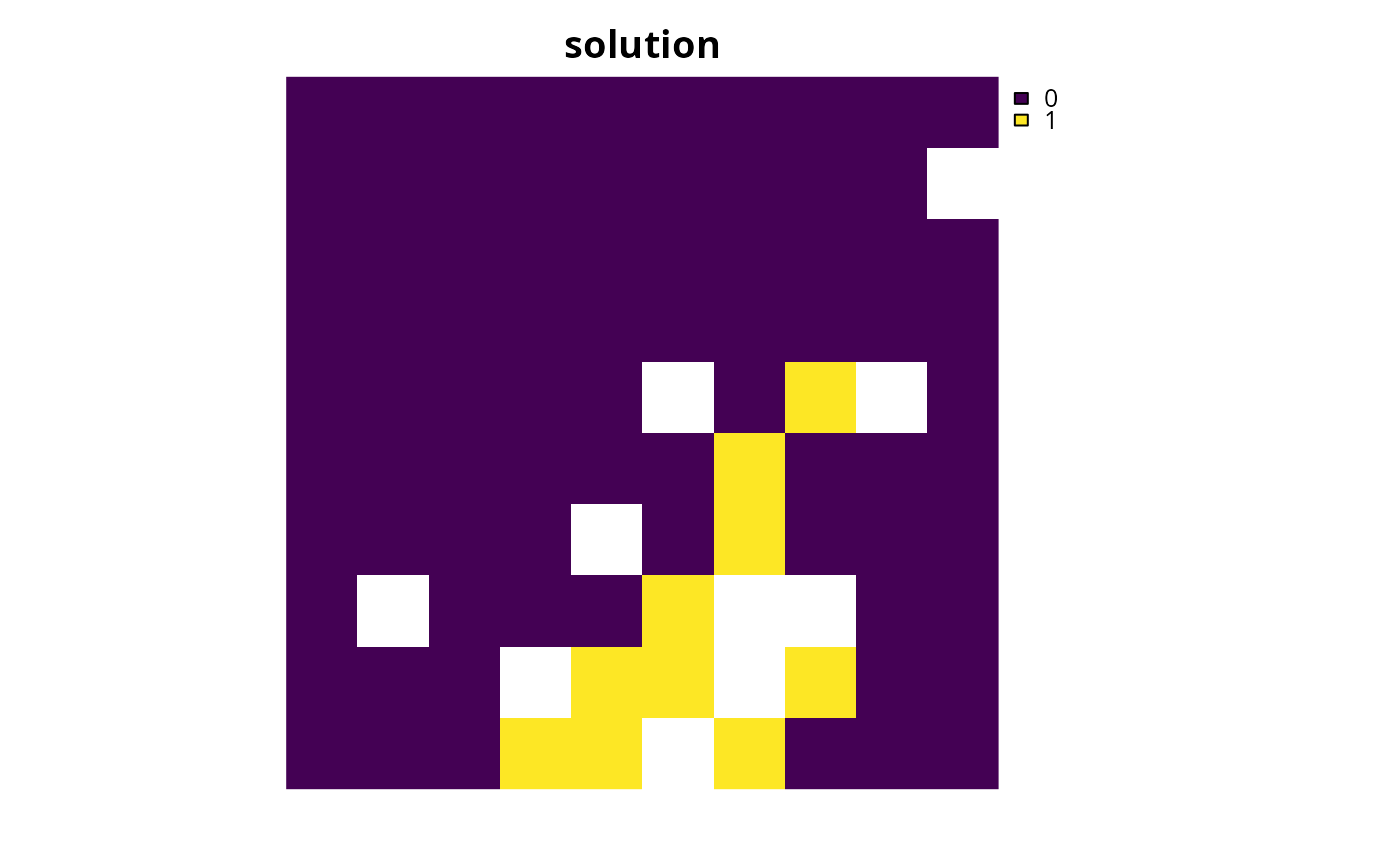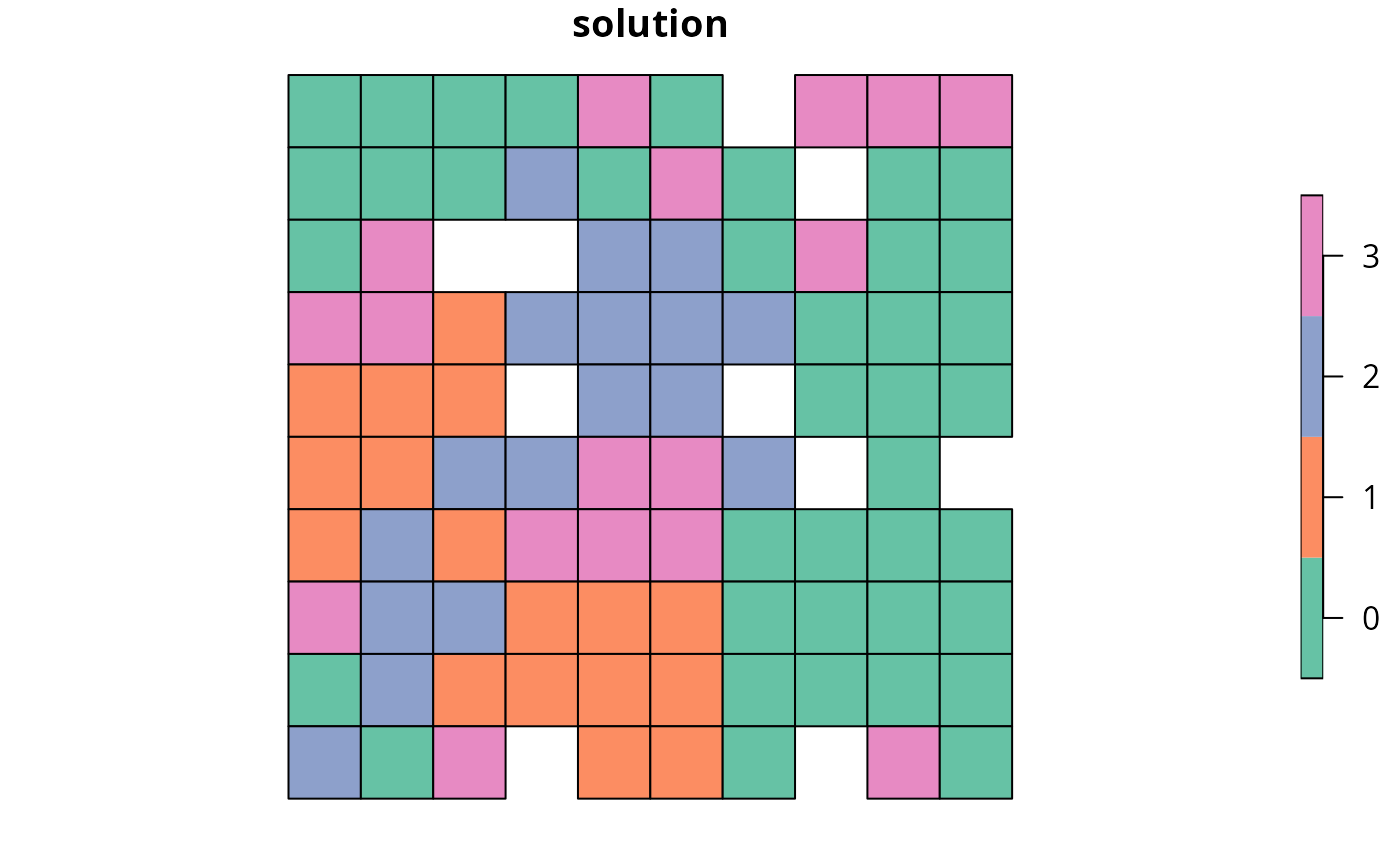Calculate the connectivity held within a solution to a conservation planning problem(). This summary statistic evaluates the connectivity of a solution using pair-wise connectivity values between combinations of planning units. It is specifically designed for symmetric connectivity data.

# S4 method for ConservationProblem,ANY,ANY,matrix
eval_connectivity_summary(x, solution, zones, data)

# S4 method for ConservationProblem,ANY,ANY,Matrix
eval_connectivity_summary(x, solution, zones, data)

# S4 method for ConservationProblem,ANY,ANY,data.frame
eval_connectivity_summary(x, solution, zones, data)

# S4 method for ConservationProblem,ANY,ANY,dgCMatrix
eval_connectivity_summary(x, solution, zones, data)

# S4 method for ConservationProblem,ANY,ANY,array
eval_connectivity_summary(x, solution, zones, data)

## Arguments

x

problem() (i.e., ConservationProblem) object.

solution

numeric, matrix, data.frame, Raster, Spatial, or sf::sf() object. The argument should be in the same format as the planning unit cost data in the argument to x. See the Solution format section for more information.

zones

matrix or Matrix object describing the level of connectivity between different zones. Each row and column corresponds to a different zone in the argument to x, and cell values indicate the level of connectivity between each combination of zones. Cell values along the diagonal of the matrix represent the level of connectivity between planning units allocated to the same zone. Cell values must lay between 1 and -1, where negative values favor solutions with weak connectivity. The default argument to zones is an identity matrix (i.e., a matrix with ones along the matrix diagonal and zeros elsewhere), so that planning units are only considered to be connected when they are allocated to the same zone. This argument is required when working with multiple zones and the argument to data is a matrix or Matrix object. If the argument to data is an array or data.frame with data for multiple zones (e.g., using the "zone1" and "zone2" column names), this argument must explicitly be set to NULL otherwise an error will be thrown.

data

matrix, Matrix, data.frame, or array object containing connectivity data. The connectivity values correspond to the strength of connectivity between different planning units. Thus connections between planning units that are associated with higher values are more favorable in the solution. See the Data format section for more information.

## Value

tibble::tibble() object describing the connectivity of the solution. It contains the following columns:

summary

character description of the summary statistic. The statistic associated with the "overall" value in this column is calculated using the entire solution (including all management zones if there are multiple zones). If multiple management zones are present, then summary statistics are also provided for each zone separately (indicated using zone names).

connectivity

numeric connectivity value. Greater values correspond to solutions associated with greater connectivity. Thus conservation planning exercises typically prefer solutions with greater values.

## Details

This summary statistic is comparable to the Connectivity_In metric reported by the Marxan software (Ball et al. 2009). It is calculated using the same equations used to penalize solutions with connectivity data (i.e., add_connectivity_penalties()). Specifically, it is calculated as the sum of the pair-wise connectivity values in the argument to data, weighted by the value of the planning units in the solution.

## Solution format

Broadly speaking, the argument to solution must be in the same format as the planning unit data in the argument to x. Further details on the correct format are listed separately for each of the different planning unit data formats:

x has numeric planning units

The argument to solution must be a numeric vector with each element corresponding to a different planning unit. It should have the same number of planning units as those in the argument to x. Additionally, any planning units missing cost (NA) values should also have missing (NA) values in the argument to solution.

x has matrix planning units

The argument to solution must be a matrix vector with each row corresponding to a different planning unit, and each column correspond to a different management zone. It should have the same number of planning units and zones as those in the argument to x. Additionally, any planning units missing cost (NA) values for a particular zone should also have a missing (NA) values in the argument to solution.

x has Raster planning units

The argument to solution be a Raster object where different grid cells (pixels) correspond to different planning units and layers correspond to a different management zones. It should have the same dimensionality (rows, columns, layers), resolution, extent, and coordinate reference system as the planning units in the argument to x. Additionally, any planning units missing cost (NA) values for a particular zone should also have missing (NA) values in the argument to solution.

x has data.frame planning units

The argument to solution must be a data.frame with each column corresponding to a different zone, each row corresponding to a different planning unit, and cell values corresponding to the solution value. This means that if a data.frame object containing the solution also contains additional columns, then these columns will need to be subsetted prior to using this function (see below for example with sf::sf() data). Additionally, any planning units missing cost (NA) values for a particular zone should also have missing (NA) values in the argument to solution.

x has Spatial planning units

The argument to solution must be a Spatial object with each column corresponding to a different zone, each row corresponding to a different planning unit, and cell values corresponding to the solution value. This means that if the Spatial object containing the solution also contains additional columns, then these columns will need to be subsetted prior to using this function (see below for example with sf::sf() data). Additionally, the argument to solution must also have the same coordinate reference system as the planning unit data. Furthermore, any planning units missing cost (NA) values for a particular zone should also have missing (NA) values in the argument to solution.

x has sf::sf() planning units

The argument to solution must be a sf::sf() object with each column corresponding to a different zone, each row corresponding to a different planning unit, and cell values corresponding to the solution value. This means that if the sf::sf() object containing the solution also contains additional columns, then these columns will need to be subsetted prior to using this function (see below for example). Additionally, the argument to solution must also have the same coordinate reference system as the planning unit data. Furthermore, any planning units missing cost (NA) values for a particular zone should also have missing (NA) values in the argument to solution.

## Data format

The argument to data can be specified using several different formats.

data as a matrix/Matrix object

where rows and columns represent different planning units and the value of each cell represents the strength of connectivity between two different planning units. Cells that occur along the matrix diagonal are treated as weights which indicate that planning units are more desirable in the solution. The argument to zones can be used to control the strength of connectivity between planning units in different zones. The default argument for zones is to treat planning units allocated to different zones as having zero connectivity.

data as a data.frame object

containing the fields (columns) "id1", "id2", and "boundary". Here, each row denotes the connectivity between a pair of planning units (per values in the "id1" and "id2" columns) following the Marxan format. If the argument to x contains multiple zones, then the columns "zone1" and "zone2" can optionally be provided to manually specify the connectivity values between planning units when they are allocated to specific zones. If the columns "zone1" and "zone2" are present, then the argument to zones must be NULL.

data as an array object

containing four-dimensions where cell values indicate the strength of connectivity between planning units when they are assigned to specific management zones. The first two dimensions (i.e., rows and columns) indicate the strength of connectivity between different planning units and the second two dimensions indicate the different management zones. Thus the data[1, 2, 3, 4] indicates the strength of connectivity between planning unit 1 and planning unit 2 when planning unit 1 is assigned to zone 3 and planning unit 2 is assigned to zone 4.

See summaries for an overview of all functions for summarizing solutions. Also, see add_connectivity_penalties() to penalize solutions with low connectivity.

Other summaries: eval_asym_connectivity_summary(), eval_boundary_summary(), eval_cost_summary(), eval_feature_representation_summary(), eval_n_summary(), eval_target_coverage_summary()

## Examples

# \dontrun{
# set seed for reproducibility
set.seed(500)

data(sim_pu_raster, sim_pu_sf, sim_features,
sim_pu_zones_sf, sim_features_zones)

# build minimal conservation problem with raster data
p1 <- problem(sim_pu_raster, sim_features) %>%

# solve the problem
s1 <- solve(p1)

# print solution
print(s1)
#> class      : RasterLayer
#> dimensions : 10, 10, 100  (nrow, ncol, ncell)
#> resolution : 0.1, 0.1  (x, y)
#> extent     : 0, 1, 0, 1  (xmin, xmax, ymin, ymax)
#> crs        : NA
#> source     : memory
#> names      : layer
#> values     : 0, 1  (min, max)
#>

# plot solution
plot(s1, main = "solution", axes = FALSE, box = FALSE)# simulate a connectivity matrix to describe the relative strength
# of connectivity between different planning units
# for brevity, we will use cost data here so that pairs
# of adjacent planning units with higher cost values will have a
# higher connectivity value
cm1 <- connectivity_matrix(sim_pu_raster, sim_pu_raster)

# calculate connectivity associated with the solution
r1 <- eval_connectivity_summary(p1, s1, data = cm1)
print(r1)
#> # A tibble: 1 × 2
#>   summary connectivity
#>   <chr>          <dbl>
#> 1 overall         198.

# build multi-zone conservation problem with polygon (sf) data
p2 <- problem(sim_pu_zones_sf, sim_features_zones,
cost_column = c("cost_1", "cost_2", "cost_3")) %>%
add_relative_targets(matrix(runif(15, 0.1, 0.2), nrow = 5,
ncol = 3)) %>%

# solve the problem
s2 <- solve(p2)

# print first six rows of the attribute table
#> Simple feature collection with 6 features and 9 fields
#> Geometry type: POLYGON
#> Dimension:     XY
#> Bounding box:  xmin: 0 ymin: 0.9 xmax: 0.6 ymax: 1
#> CRS:           NA
#>     cost_1   cost_2   cost_3 locked_1 locked_2 locked_3 solution_1_zone_1
#> 1 215.8638 183.3344 205.4113    FALSE    FALSE    FALSE                 0
#> 2 212.7823 189.4978 209.6404    FALSE    FALSE    FALSE                 0
#> 3 207.4962 193.6007 215.4212     TRUE    FALSE    FALSE                 0
#> 4 208.9322 197.5897 218.5241    FALSE    FALSE    FALSE                 0
#> 5 214.0419 199.8033 220.7100    FALSE    FALSE    FALSE                 0
#> 6 213.7636 203.1867 224.6809    FALSE    FALSE    FALSE                 0
#>   solution_1_zone_2 solution_1_zone_3                       geometry
#> 1                 0                 0 POLYGON ((0 1, 0.1 1, 0.1 0...
#> 2                 0                 1 POLYGON ((0.1 1, 0.2 1, 0.2...
#> 3                 0                 0 POLYGON ((0.2 1, 0.3 1, 0.3...
#> 4                 0                 1 POLYGON ((0.3 1, 0.4 1, 0.4...
#> 5                 0                 1 POLYGON ((0.4 1, 0.5 1, 0.5...
#> 6                 0                 0 POLYGON ((0.5 1, 0.6 1, 0.6...

# create new column representing the zone id that each planning unit
# was allocated to in the solution
s2$solution <- category_vector( s2[, c("solution_1_zone_1", "solution_1_zone_2", "solution_1_zone_3")]) s2$solution <- factor(s2$solution) # plot solution plot(s2[, "solution"])# simulate connectivity matrix # here, we will add a new column to sim_pu_zones_sf with # randomly simulated values and create a connectivity matrix # based on the average simulated values of adjacent planning units sim_pu_zones_sf$con <- runif(nrow(sim_pu_zones_sf))
cm2 <- connectivity_matrix(sim_pu_zones_sf, "con")

# calculate connectivity associated with the solution
r2 <- eval_connectivity_summary(
p2, s2[, c("solution_1_zone_1", "solution_1_zone_2", "solution_1_zone_3")],
data = cm2)
print(r2)
#> # A tibble: 4 × 2
#>   summary connectivity
#>   <chr>          <dbl>
#> 1 overall        2.06
#> 2 zone_1         0.775
#> 3 zone_2         0.516
#> 4 zone_3         0.771

# }